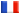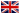# Séminaire de Cryptographie

## Pascal Paillier### Can RSA keys be instance-malleable?

We focus on two new number-theoretic problems of major importance for RSA and factoring-based cryptosystems. An RSA key generator Gen(1^k) = (n, e) is malleable when factoring n is easier when given access to a factoring oracle for other keys (n', e')!= (n, e) output by Gen. Gen is instance-malleable when it is easier to extract e-th roots mod n given an e'-th root extractor mod n' for (n', e') != (n , e) output by Gen. Instance-non-malleable generators are of prime importance for practical RSA-based systems (RSA-PSS, RSA-OAEP, etc) because their security can be shown not to be equivalent to RSA in the standard model, in contradiction with the random oracle heuristic. We investigate the malleability and instance-malleability of popular RSA key generators such as textbook RSA and low-exponent RSA and question the existence of non-trivial malleable RSA instances.